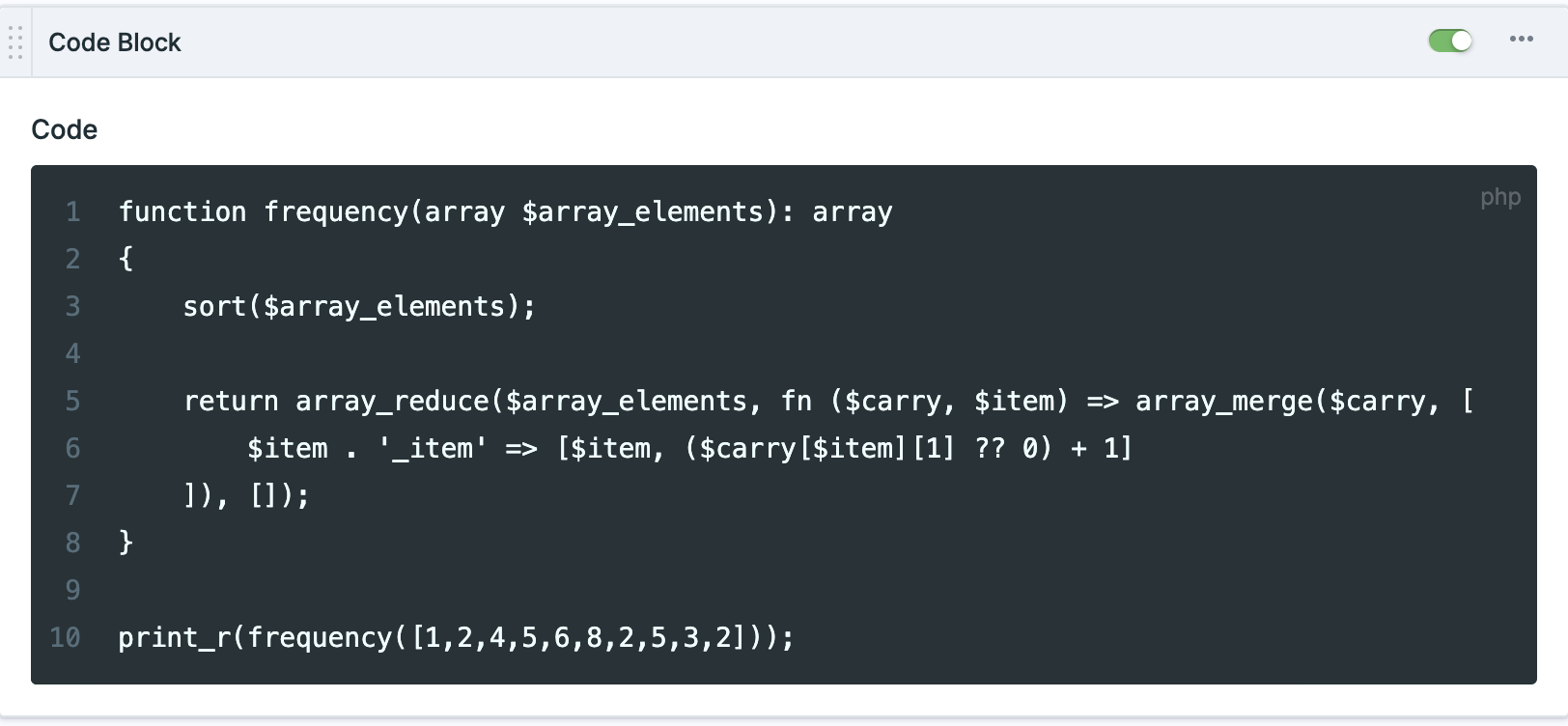# Getting started with counting and sorting numbers in PHP

Have you ever needed to count the number of times each integer appears in an array? This is a common problem that can be solved using a simple algorithm. In this post, we will walk through an efficient way to solve this problem and provide sample code in PHP.Suppose we have an array of integers, such as [1, 2, 4, 5, 6, 8, 2, 5, 3, 2]. We want to count the number of times each integer appears in the array, and then return the results in ascending order.

To solve this problem, we can create a method that takes an array of integers as an argument. The first thing we need to do is to iterate through the array and count the frequency of each number. We can do this using a dictionary, where we store each number as the key and its frequency as the value. Once we have counted the frequencies of all the numbers, we can create a new array where we store each number and its frequency as a pair of values.

To sort the array of pairs in ascending order, we can use the PHP `sort` function. Here is an example of how we could implement this function:

``````function frequency(array \$array_elements): array
{
sort(\$array_elements);

return array_reduce(\$array_elements, fn (\$carry, \$item) => array_merge(\$carry, [
\$item . '_item' => [\$item, (\$carry[\$item] ?? 0) + 1]
]), []);
}

print_r(frequency([1,2,4,5,6,8,2,5,3,2]));``````

First, the function uses the `sort` function to sort the input array in ascending order. This ensures that the returned array will also be in ascending order, which is specified in the problem statement.

Next, the function uses the `array_reduce` function to reduce the input array to a single value. This function takes two arguments: the input array and a callback function that specifies how to reduce the array to a single value. In this case, the callback function uses the `array_merge` function to combine the current array of frequencies with a new array containing the frequency of the current element.

The callback function uses the `\$carry` variable to store the current array of frequencies, and the `\$item` variable to store the current element being processed. The function first checks if the current element is already in the `\$carry` array, and if it is, it increments the frequency of that element by 1. If the element is not in the `\$carry` array, the function adds a new entry with a frequency of 1.

Finally, the `frequency` function returns the resulting array of frequencies, which contains the frequency of each element in the input array.

If you want to get some examples of this function for using laravel collection you can contact me by email at [email protected] or on Twitter.